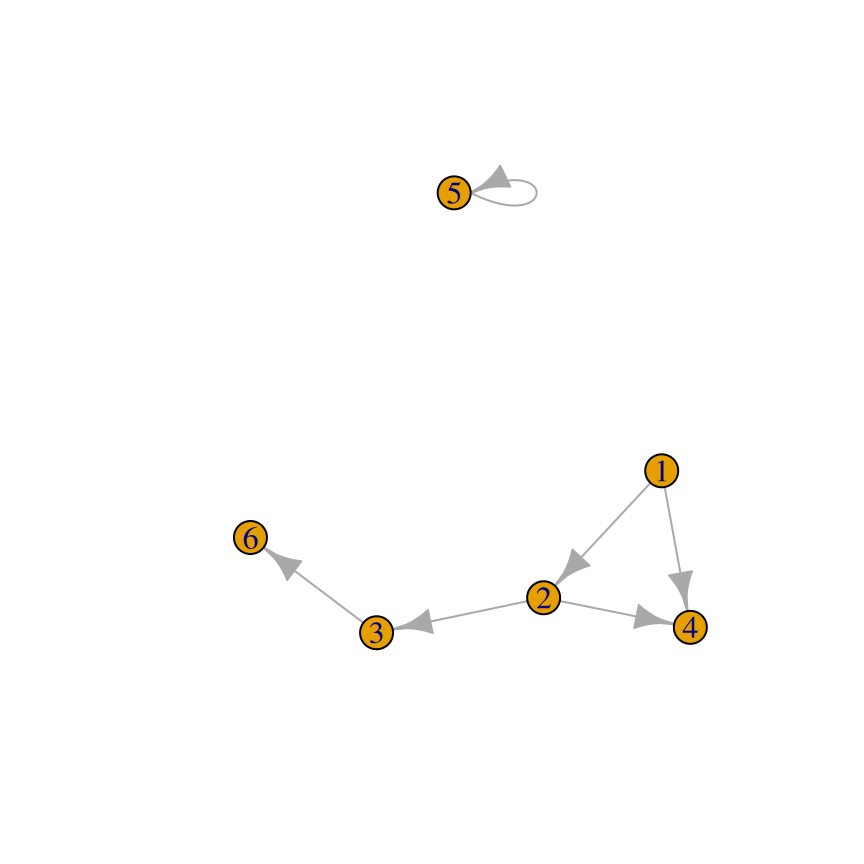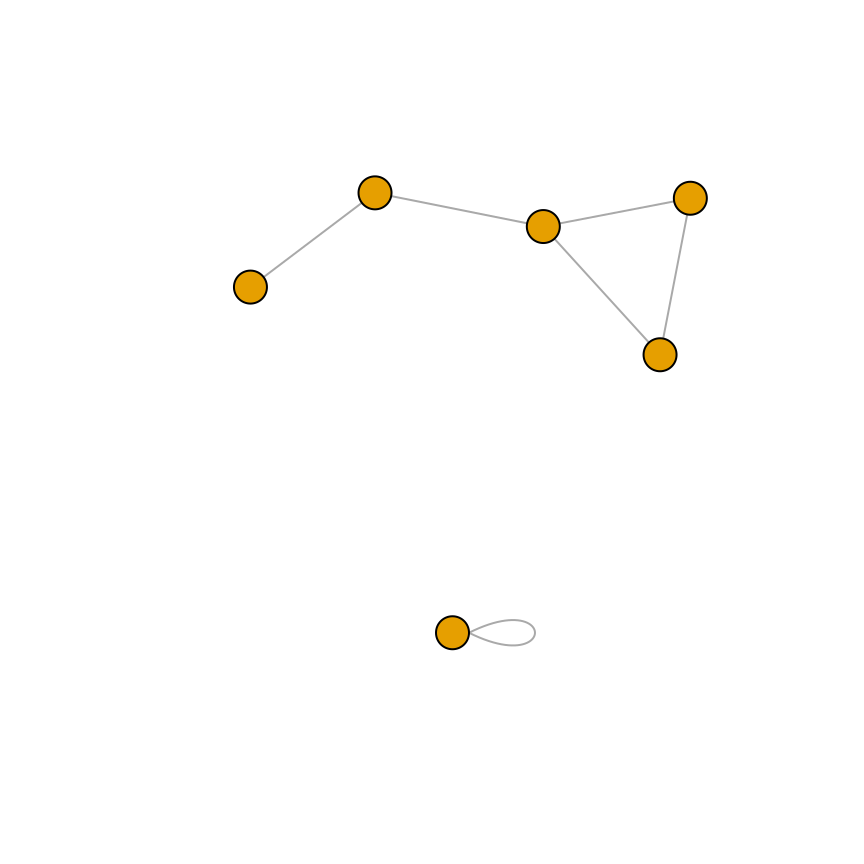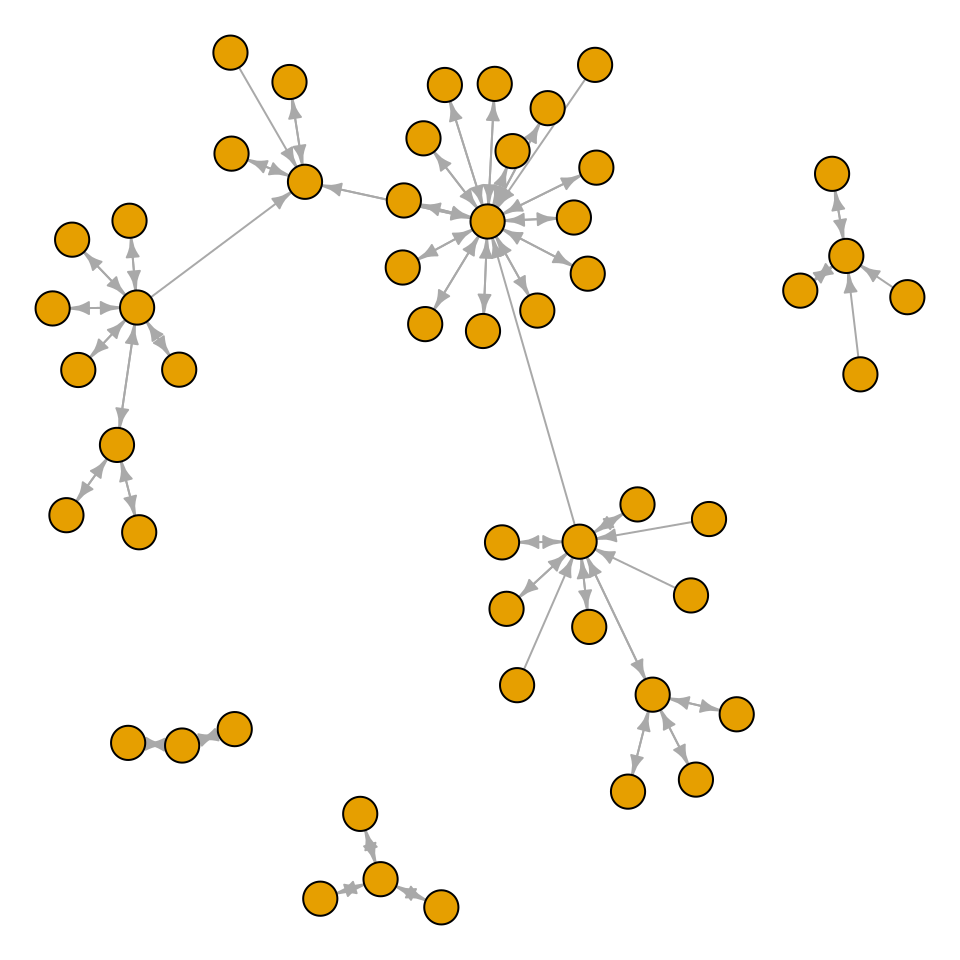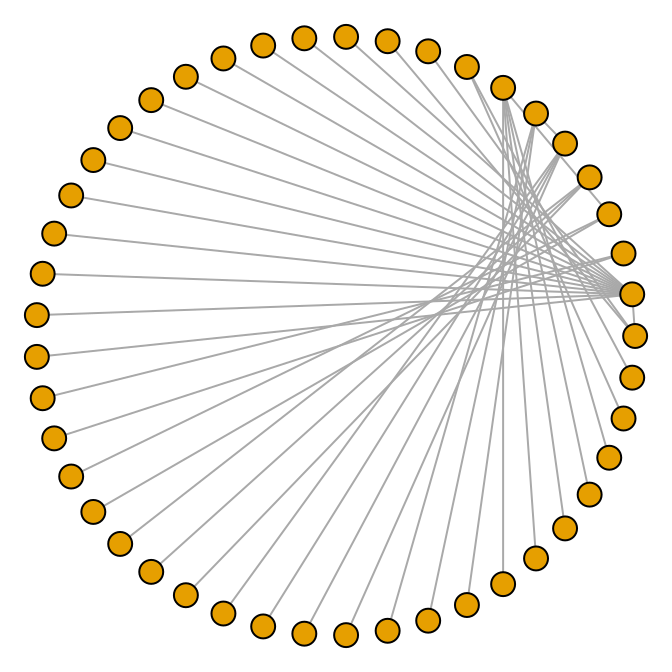## 13.4 Creating a Network Graph

### 13.4.1 Problem

You want to create a network graph.

### 13.4.2 Solution

Use the igraph package. To create a graph, pass a vector containing pairs of items to `graph()`, then plot the resulting object (Figure 13.7):

``````# May need to install first, with install.packages("igraph")
library(igraph)

# Specify edges for a directed graph
gd <- graph(c(1,2, 2,3, 2,4, 1,4, 5,5, 3,6))
plot(gd)

# For an undirected graph
gu <- graph(c(1,2, 2,3, 2,4, 1,4, 5,5, 3,6), directed = FALSE)
# No labels
plot(gu, vertex.label = NA)``````Figure 13.7: A directed graph (left); An undirected graph, with no vertex labels (right)

This is the structure of each of the graph objects:

``````gd
#> IGRAPH 8fdbbc9 D--- 6 6 --
#> + edges from 8fdbbc9:
#>  1->2 2->3 2->4 1->4 5->5 3->6
gu
#> IGRAPH 1dffb91 U--- 6 6 --
#> + edges from 1dffb91:
#>  1--2 2--3 2--4 1--4 5--5 3--6``````

### 13.4.3 Discussion

In a network graph, the position of the nodes is unspecified by the data, and they’re placed randomly. To make the output repeatable, you can set the random seed before making the plot. You can try different random numbers until you get a result that you like:

``````set.seed(229)
plot(gu)``````

It’s also possible to create a graph from a data frame. The first two columns of the data frame are used, and each row specifies a connection between two nodes. In the next example (Figure 13.8), we’ll use the `madmen2` data set, which has this structure. We’ll also use the Fruchterman-Reingold layout algorithm. The idea is that all the nodes have a magnetic repulsion from one another, but the edges between nodes act as springs, pulling the nodes together:

``````library(gcookbook) # For the data set
#>                       Name1          Name2
#> 1               Abe Drexler    Peggy Olson
#> 2                   Allison     Don Draper
#> 3               Arthur Case   Betty Draper
#>  ...<81 more rows>...
#> 85                    Vicky Roger Sterling
#> 86                 Waitress     Don Draper
#> 87 Woman at the Clios party     Don Draper
# Create a graph object from the data set
# Remove unnecessary margins
par(mar = c(0, 0, 0, 0))
plot(g, layout = layout.fruchterman.reingold, vertex.size = 8,
edge.arrow.size = 0.5, vertex.label = NA)``````Figure 13.8: A directed graph from a data frame, with the Fruchterman-Reingold algorithm

It’s also possible to make a directed graph from a data frame. The `madmen` data set has only one row for each pairing, since direction doesn’t matter for an undirected graph. This time we’ll use a circle layout (Figure 13.9):

``````g <- graph.data.frame(madmen, directed = FALSE)
par(mar = c(0, 0, 0, 0))  # Remove unnecessary margins
plot(g, layout = layout.circle, vertex.size = 8, vertex.label = NA)``````Figure 13.9: A circular undirected graph from a data frame

For more information about the available output options, see `?plot.igraph`. Also see `?igraph::layout` for layout options.